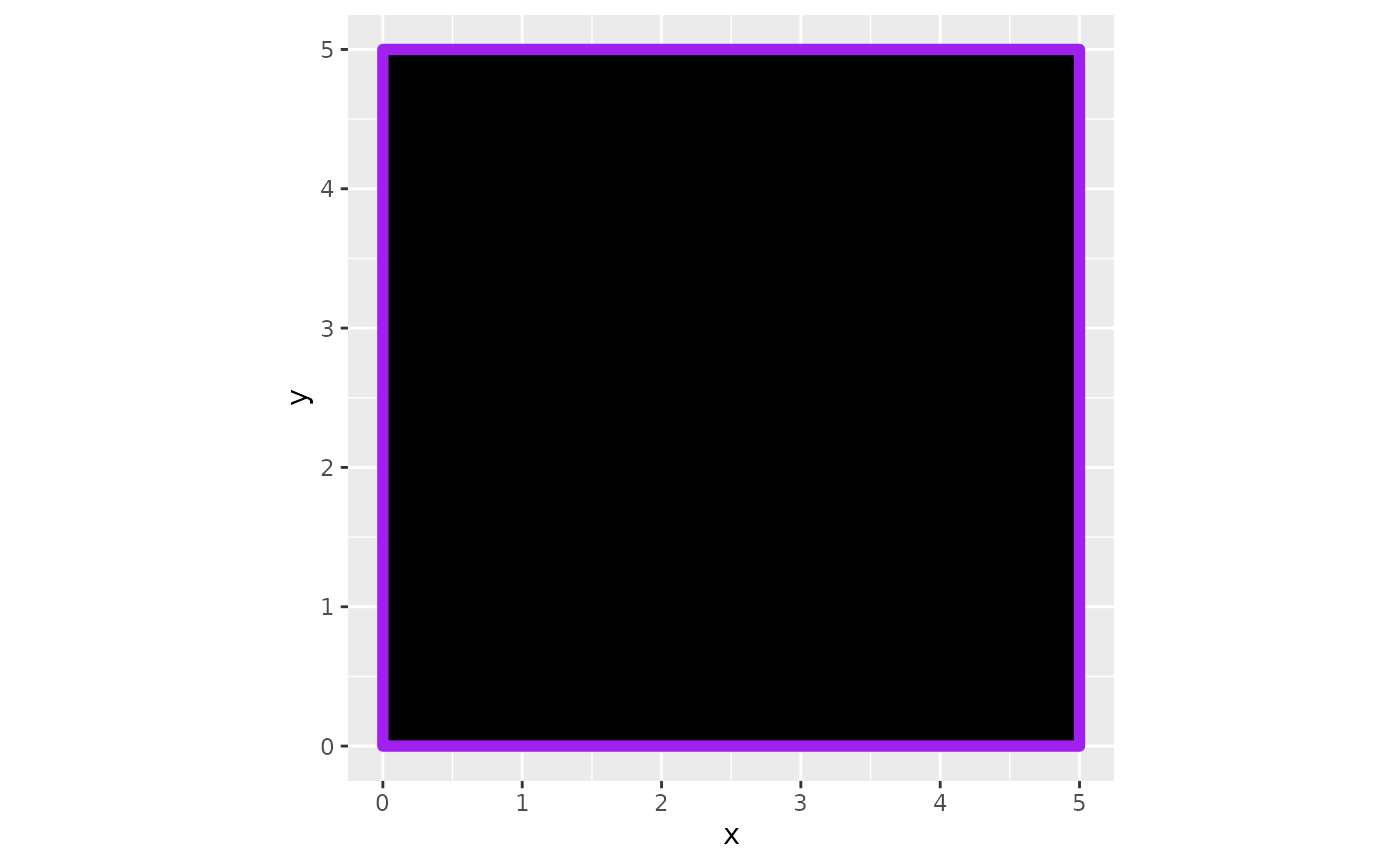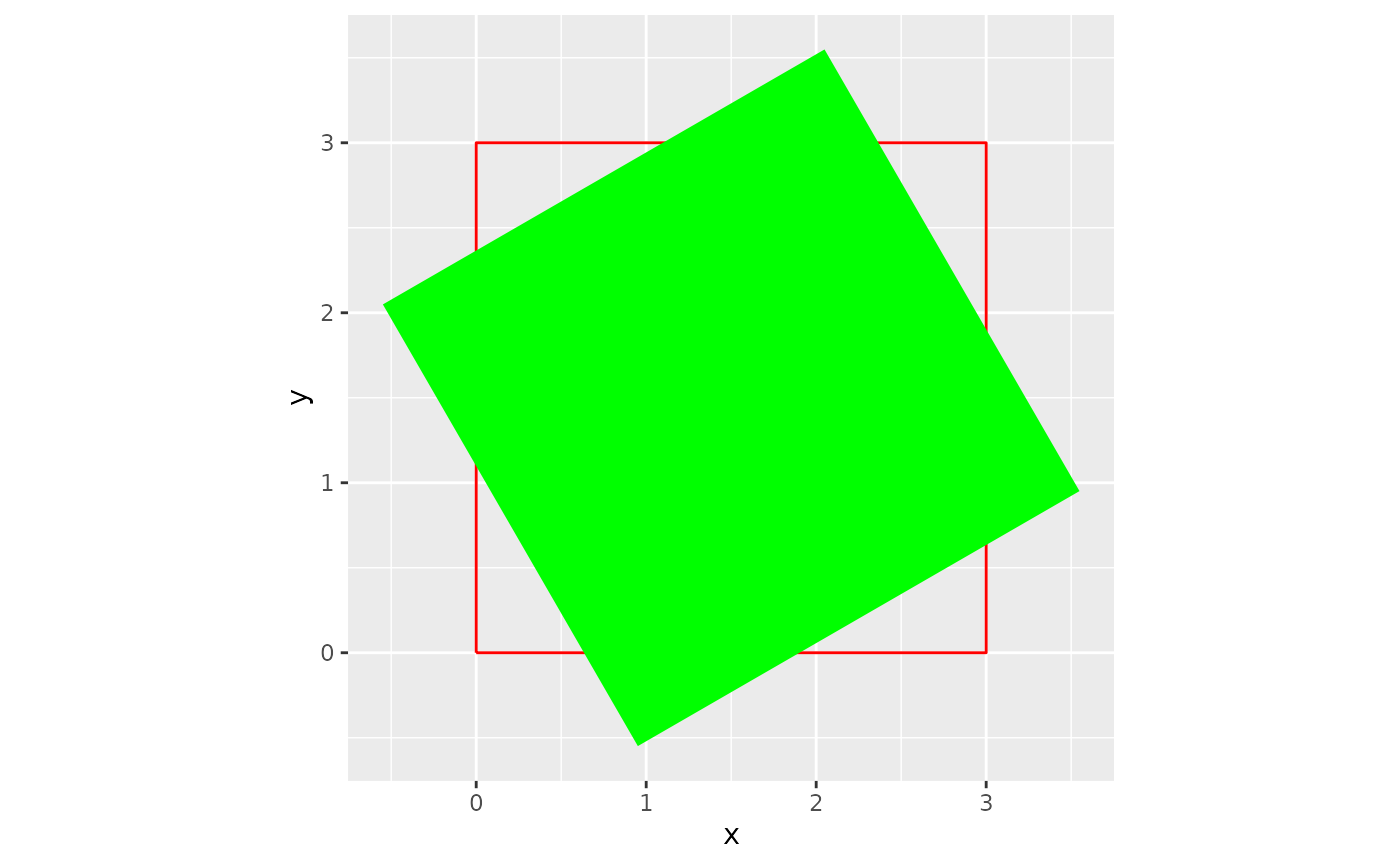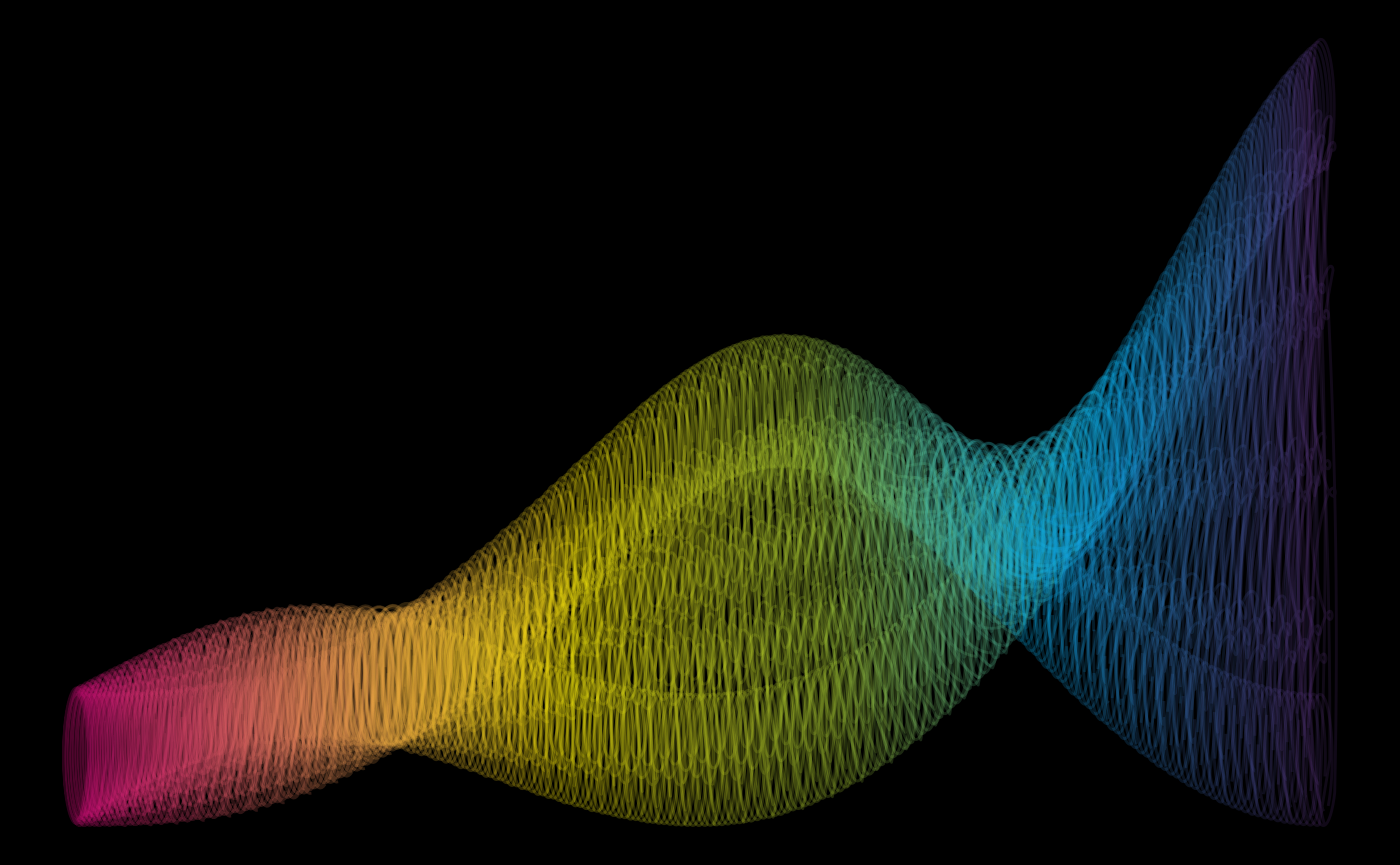## Brief Examples

aRtpack can be used to create specified dataframes that will map art when fed into ggplot2 functions:

For example, square_data() creates a data frame that maps a square on to a ggplot:

library(ggplot2)
library(artpack)

# Use the function to create a data frame #
df_square <-
square_data(
x = 0,
y = 0,
size = 5,
color = "purple",
fill = "black"
)

# Feed it into a ggplot #
df_square |>
ggplot(aes(x = x, y = y)) +
geom_polygon(
fill = df_square$fill, color = df_square$color,
linewidth = 2
) +
coord_equal()rotator will mathematically “rotate” existing data points in a data frame:

library(ggplot2)
library(artpack)

original_square <- data.frame(
x = c(0, 3, 3, 0, 0),
y = c(0, 0, 3, 3, 0)
)

rotated_square <- rotator(
data = original_square,
x = x,
y = y,
angle = 120,
anchor = "center"
)

ggplot() +
geom_path(
data = original_square,
aes(x, y),
color = "red"
) +
geom_polygon(
data = rotated_square,
aes(x, y),
fill = "green"
) +
coord_equal()aRtpack functions are designed to be used in any part of your workflow. Experiment for some cool results:

library(ggplot2)
library(purrr)
library(dplyr)
#>
#> Attaching package: 'dplyr'
#> The following objects are masked from 'package:stats':
#>
#>     filter, lag
#> The following objects are masked from 'package:base':
#>
#>     intersect, setdiff, setequal, union
library(tibble)
library(artpack)

# Create a base square #
square <- square_data(x = 0, y = 0, size = 1, group_var = TRUE)

# Create square specs to be iterated on #
n_square <- 50
scaler <- seq(1, 5, length = n_square)
fills <- art_pals("imagination", n = n_square)
angles <- seq(0, 360, length = n_square)
group_n <- group_numbers(1:n_square)

# Add a random transformation for a little razzle dazzle ✨
theta <- seq(0, 2 * pi, length = 250)

list_opts <- list(
scaler,
fills,
angles,
group_n
)

df <- pmap(list_opts, ~ rotator(
square |>
mutate(
x = (x + ..1),
y = (y + ..1),
fill = ..2,
group = paste0(group, ..4)
),
x = x, y = y, angle = ..3
)) |>
list_rbind() |>
mutate(
x = x * cos(theta) + x,
y = y * sin(theta)
)

df |>
ggplot(aes(x = x, y = y, group = group)) +
theme_void() +
theme(plot.background = element_rect(fill = "#000000")) +
geom_polygon(
fill = df\$fill,
color = "#000000",
alpha = .5
) +
coord_equal(expand = FALSE)